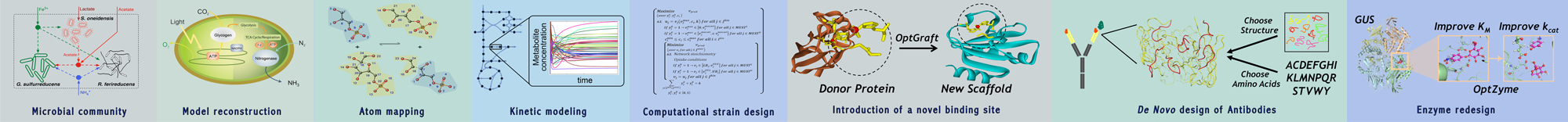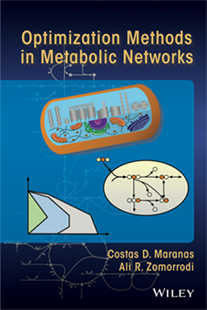Thank you for taking interest in our book. In this page, you can find a summary of each chapter, links to the Wiley Online Library and source codes for some of the examples in the book. The solutions for exercise problems at the end of each chapter are available upon request (costas@psu.edu) to course instructors.## Optimization Methods in Metabolic Networks

Dr. Costas D. Maranas and Dr. Ali R. Zomorrodi published new book that explores optimization concepts in metabolic networks.

This chapter reviews the fundamentals of mathematical optimization and modeling. It starts with a biological network inference problem as a prototype example to highlight the basic steps of formulating an optimization problem. This is followed by a review of some basic mathematical concepts and definitions such as set and function properties and convexity analysis.

Links: Wiley Online Library

This chapter introduces the basic concepts of linear programming (LP), discusses the logic of the Simplex method for solving LP problems, and introduces LP duality theory. Furthermore, useful transformation techniques to convert a number of nonlinear optimization problems to an equivalent LP representation are introduced. These include optimization problems containing absolute values, min–max optimization problems, and linear fractional programming representations.

Links: Wiley Online Library

This chapter starts with a brief introduction of metabolism and highlights basic concepts underpinning mathematical modeling of metabolism along with a description of genome-scale metabolic (GSM) models. Subsequently, flux balance analysis (FBA) for metabolic networks is presented. Finally, a number of popular of applications of FBA based on linear programming (LP) including the modeling of gene/reaction knockouts, calculation of maximum theoretical yield for a product of interest, flux variability analysis, and flux coupling analysis of metabolic networks are discussed.

This chapter describes how to model logic statements in optimization problems using binary variables followed by a brief overview of the branch-and-bound procedure for solving mixed-integer linear programming (MILP) problems. Efficient formulation strategies for solving MILP problems are also discussed. This is followed by an MILP problem in metabolic networks addressing the identification of minimal reactions sets supporting growth under different substrate uptake scenarios.

This chapter discusses how to systematically assess the allowable reaction directionalities and eliminate thermodynamically infeasible cycles in metabolic network reconstructions using systematic optimization-based methods. In particular, thermodynamic-based metabolic flux analysis (TMFA) and Loopless constraint-based reconstruction and analysis (ll-COBRA) procedures are discussed.

Links: Wiley Online Library

This chapter outlines basic concepts and methods based on linear programming (LP) and mixed-integer LP (MILP) for testing and correcting metabolic models. In particular, procedures GapFind and GapFill help pinpoint and bridge gaps in metabolic models, whereas procedure GrowMatch reconciles model growth prediction inconsistencies with experimental data. In addition, best practices for confirming or refuting model correction suggestions are discussed.

Links: Wiley Online Library

This chapter focuses on mixed-integer linear programming (MILP)-based approaches for searching through a reaction list and/or genome-scale metabolic model for pathways toward a target product. It starts with introducing an MILP procedure that integrates path-finding algorithms in graphs with flux balance analysis (FBA) to identify shortest reaction paths between a source and a target metabolite. Subsequently, an MILP-based optimization procedure is introduced for identifying nonnative reactions to add to a metabolic network to enable the production of a target product. Finally, a two-step procedure is presented, which first optimizes the overall stoichiometry of conversion of a source to a target metabolite while taking into account coreactants/coproducts and maintaining thermodynamic feasibility, and next identifies intervening reactions from a database enabling the optimized stoichiometric ratios.

Links: Wiley Online Library

This chapter introduces some of the core computational strain design protocols based on bilevel and MILP formulations. These procedures identify alternative sets of required genetic interventions (gene knockouts/ins/ups/downs) redirecting the metabolic flow toward a product of interest. By using the popular computational strain design protocol, OptKnock, as a prototype example the algorithmic details for formulating and solving bilevel optimization problems are presented along with a cursory treatment of other strain design procedures.

This chapter outlines the fundamentals of unconstrained and constrained nonlinear programming and popular solution procedures. Optimality conditions for both unconstrained and constrained optimization problems are discussed. Steepest descent, Newton's, quasi-Newton, and conjugate gradients methods for unconstrained problems and sequential quadratic programming and generalized reduced gradients methods for constrained problems are presented. The chapter also introduces Lagrangian duality theory for NLP problems.

Links: Wiley Online Library

This chapter presents three examples of nonlinear optimization in the analysis of metabolic networks. The first example replaces the biomass flux maximization in flux balance analysis (FBA) with a nonlinear objective function enforcing the minimization of metabolic adjustment (MOMA). The second example introduces kinetic expressions to quantify the flux of some of the reactions in a stoichiometric metabolic model. The last example considers a least squares minimization problem arising in metabolic flux elucidation consistent with isotope labeling data (MFA) involving a nonlinear objective function and constraints.

This chapter introduces 0–1 mixed-integer nonlinear programming (MINLP) problems and outlines a number of popular solution procedures including generalized Benders’ decomposition, outer approximation, and outer approximation with equality constraints. This is followed by an MINLP-based extension of the OptKnock procedure (Chapter 8) that allows for kinetic descriptions for some of the reactions in a stoichiometric metabolic model.

Links: Wiley Online Library

A tutorial for using GAMS is provided in this chapter.